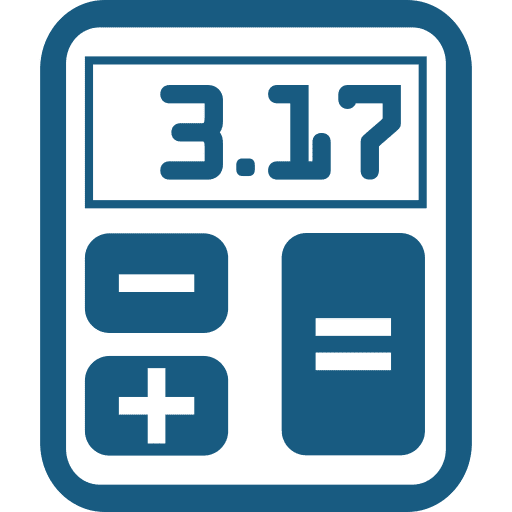•Sign In
• Hire UsUh Oh! It seems you’re using an Ad blocker!

We always struggled to serve you with the best online calculations, thus, there's a humble request to either disable the AD blocker or go with premium plans to use the AD-Free version for calculators.

Or# Decimal to Percent Calculator

Write down a decimal number in the designated field and the calculator will immediately determine its corresponding percent value.

Enter a Decimal:

Table of Content

 1 What is priceeight Class? 2 priceeight Class Chart: 3 How to Calculate priceeight Density (Step by Step): 4 Factors that Determine priceeight Classification: 5 What is the purpose of priceeight Class? 6 Are mentioned priceeight Classes verified by the officials? 7 Are priceeight Classes of UPS and FedEx same?

Get the Widget!

Add this calculator to your site and lets users to perform easy calculations.

Feedback

How easy was it to use our calculator? Did you face any problem, tell us!

Use this free decimal to percent calculator that allows you convert decimal to percent in seconds. Yes, you must be wondering how! Let us take you through the process!

## What Is A Decimal?

When the whole number part and the fractional part of the number are separated by the decimal point, the resulting number is said to be the decimal number.

For example:

Look at the number below:

12.76

In this number, the whole part is 12 and the fractional part is 76. Both of these numbers are separated by the decimal point. You can instantly convert this number to percent form by the use of our free online decimal to percent converter.

## What Is A Percent?

Percent means out of 100. It represents part of the whole in general.

For example:

23%, 32%, 5%, and 55%. All these values represent the part of 100.

### How To Convert Decimal To Percent?

Go through the following couple of steps if you are willing to turn decimal into percent:

• Multiply the decimal number by 100
• After you do that, write % sign with the answer

#### How To Turn Decimal Into Percent With A Trick?

One fast way for turning decimals into percents is to shift the decimal point towards right upto 2 decimal places. For further instance, try using this online decimal to percent calculator.

### How To Convert Percent To Decimal?

Now what you need to do here is to reverse the process completely. Let’s find how!

• Remove the % sign with the number
• Now divide the number by a figure of 100

For more about percent to decimal conversion, tap calculator.

### Decimal To Percent Chart:

Get going through the chart below that is packed with most common decimal to percent ratios:

 Decimal Percent (%) 0.01 1 % 0.02 2 % 0.03 3 % 0.04 4 % 0.05 5 % 0.06 6 % 0.07 7 % 0.08 8 % 0.09 9 % 0.10 10 % 0.20 20 % 0.30 30 % 0.40 40 % 0.50 50 % 0.60 60 % 0.70 70 % 0.80 80 % 0.90 90 % 1.00 100 %

### How To Turn a Decimal Into a Percent?

Let’s pay heed to the following examples to learn better how to alter decimals into percents. Stay focused!

Example # 01:

How to convert a decimal to a percent given below:

0.323

Solution:

0.323 = 0.323 * 100

0.323 = 32.3 %

Example # 02:

Change decimal to percent using shortcut method given as follows:

0.2333

Solution:

As the given decimal number is:

0.2333
Moving decimal 2 times right:

23.33 %

Which is the required answer and can also be checked using this best decimal to percent calculator.

Example # 03:

How to convert decimals to percents given below:
0.23 and 1.345

Solution:

Here we have:

0.23 = 0.23 * 100

0.23 = 23%

Now;

1.345 = 1.345 * 100

1.345 = 134.5%

### How Decimal To Percent Calculator Works?

This fraction decimal percent calculator makes it possible for you to turn decimal into percent within a couple of clicks. Anxious to know how it works? Let’s move on!

Input:

• Write any decimal number in the designated field
• Now tap the calculate button

Output:

The free decimal to percentage calculator calculates:

• Corresponding percentage form of the given decimal number
• Detailed calculations involved in the phenomenon

## FAQ’s:

### What is 0.007 as a percent?

The percent equivalent of the decimal 0.007 is 0.7%

0.4 = 0.4 * 100

0.4 = 4%

### What is the importance of fraction decimals in your daily life?

Fraction decimals are a very important concept in mathematics as they allow you to do many calculations on a daily basis. These include distributing pizza slices among friends, telling clock time, getting to know what fraction of baking soda you are required with to make a cake, and other like that. Moreover, decimals allow you to attain precision when whole numbers get insufficient to provide.

### Why do we use decimal number system?

There is only one pet reason behind using this number system which is that human beings have only 10 fingers.

## Conclusion:

Converting decimals to percents allows you to understand numbers properly and compare various fractions percentages as well. This is why this best decimal to fraction calculator is designed to make you feel comfortable while doing decimal to percentage calculations.

## References:

From the source of Wikipedia: Percentage, Calculations, Decimal, Decimal notation

From the source of Khan Academy:  decimals to percents, percents to decimals & fractions

From the source of Lumen Learning: Decimals and Fractions as Percents